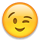# end_segments() and start_segments() function related

edited April 2021

Hello, Matthias & sirs

I found the end_segments() and start_segments() functions might re-build the sequence for the " edges object " in an edge set?

for example: ( part of the script as below)

`target = pya.Region( layout.top_cell().begin_shapes_rec(metal)).with_angle(0, 45 , False)`
(I get an edgepair set, totally 233 pairs)

`edge_1 = target.first_edges().end_segments(1000,0.0)`
(then I try to gain every end part the first_edge with 1 um length)

• then I found `target.first_edges[x]` is exactly equal to the first part of `target[x]` for all 233 object )
• but the edges sequence might be changed in `edge_1`, in my case, the dedicated `target` moved to `edge_1`, it seems random
• result: `target` = (930972 , 1809672 ; 930972 , 557960)
• result: `edge_1` = (930972 , 558960 ; 930972 , 557960)

Not sure it is normal situation, or due to edges too many ?
Sorry, I am not used to this typing interface , hope my explanation is ok to read... thanks all

Deal patterns with Klayout Python function is funnyThanks for your explanation